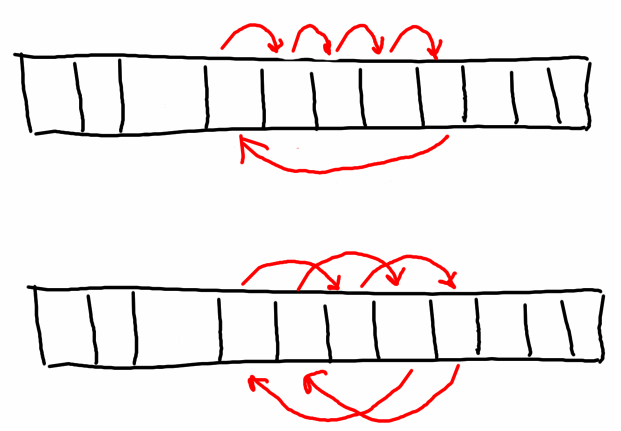In some code we needed to perform a circular shift of a part of array, like on the following picture:It's clear what to do, especially in case of one element shift but think about "optimal" algorithm that does minimal number of data movemenents.

Here is what we have came up with in C#: algorithm doing single pass over data.

```/// <summary>
/// <para>
/// Moves content of list within open range <code>[start, end)</code>.
/// <code>to</code> should belong to that range.
/// </para>
/// <para>
/// <code>list[start + (to - start + i) mod (end - start)] =
/// list[start + i]</code>,
/// where i in range<code>[0, end - start)</ code >.
/// </para>
/// </summary>
/// <typeparam name="T">An element type.</typeparam>
/// <param name="list">A list to move data withing.</param>
/// <param name="start">Start position, including.</param>
/// <param name="end">End position, not incuding.</param>
/// <param name="to">Target position.</param>
public static void CircularMove<T>(IList<T> list, int start, int end, int to)
{
var size = end - start;
var step = to - start;
var anchor = start;
var pos = start;
var item = list[pos];

for(int i = 0; i < size; ++i)
{
pos += step;

if (pos >= end)
{
pos -= size;
}

var next = list[pos];

list[pos] = item;
item = next;

if (pos == anchor)
{
pos = ++anchor;

if (pos >= end)
{
break;
}

item = list[pos];
}
}
}```
Archive
 < September 2023 >
SunMonTueWedThuFriSat
272829303112
3456789
10111213141516
17181920212223
24252627282930
1234567
Blogroll
Statistics
Total Posts: 384
This Year: 3
This Month: 0
This Week: 0